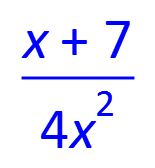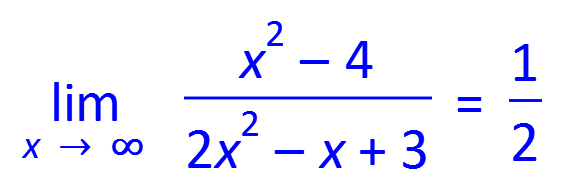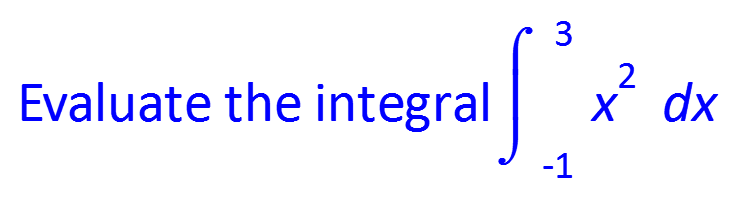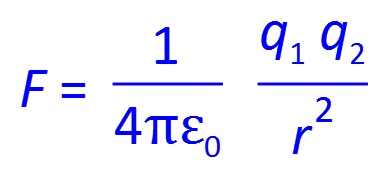# FX Equation

Type Mathematics

FX Equation lets you create equations at the speed of type. Our new FX AutoQuate technology creates an incredibly productive equation-writing environment. You can still create equations using "point and click" but common equations can be produced amazingly fast.#### Just Type

FX Equation is renowed for it's extremely high speed equation entry. Most equations can just be "typed" and FX Equation will interpret what you have typed, putting everything in the correct place. For example, typing (x+7)/4x2 will automatically produce this equation. No point and click, just type.

#### There Are Limits!

FX Equation's "type what you want" paradigm has some limits. In particular, we have limited what it can produce to be representative of the needs of secondary school mathematics and physics teachers. There are some restrictions, but not as many as you might think.

lim(x->inf) (x2-4)/(2x2-x+3) = 1/2#### Seriously Clever

Our FX Autoquate technology lets you just type and FX Equation will automatically decide which bits are equations and which bits are normal text. You don't have to "do" anything except type. This lets you stop thinking about "how" and concentrate on what you want to write.

Evaluate the integral int(-1,3) x2 dx

# Trial Versions

These trial versions work in exactly the same way as the registered versions, they just overlay a watermark over all graphics. Logging in to the software with your account details turns them into full versions. Try out all of the features in FX Draw and the new FX Online.

#### Not Just Mathematics

FX Equation is just at good at rendering the sorts of equations that physics teachers use regularly. This makes it a very useful addition to the FX SciencePack subscription.

F= 1/(4piep\0) (q\1 q\2)/r2# Trial Versions

These trial versions work in exactly the same way as the registered versions, they just overlay a watermark over all graphics. Logging in to the software with your account details turns them into full versions. Try out all of the features in the FX Science Tools.

#### Take A Look

This short video will show you how efficient FX Equation can be.

## Feature List

#### FX Autoquate

• Type mixed equations and text and have FX Autoquate format automatically.
• Advanced Bayesian analysis used to detect equations.
• Automatic formatting of text and equations using predetermined, overrideable formatting defaults.
• System designed for secondary school level mathematics.

#### Equation Entry

• Highly productive, text based equation entry system. Just type.
• Equations formatted as you type.
• Word-processor like formatting commands that apply to text and equations.
• Menu based equation entry that can be used instead of, or in addition to, text entry.

#### Equation Elements

• Lower case and upper case Greek letters.
• Automatic exponentiation of expressions such as x2
• Full complement of mathematical symbols (secondary level mathematics).
• Doublestruck symbols for number sets.
• Equation numbering.
• Short division signs.
• Integrals.
• Limits.
• Sigma and product notations.
• Scientific notation.
• Add boxes around expressions and create empty boxes.
• Permutations and combinations.
• Lowercase and uppercase vectors using a variety of notations.
• Matrices and column vectors.
• Arc over expression.
• Subscripts.
• Bar over expressions.
• Hats and dots over epxressions.
• Customisable shortcuts for complex expressions.
• Automatic formatting of composite expressions.
• Automatic formatting of mixed numerals.
• Recurring decimals.
• Greatest integer symbols/brackets.
• Definite integral bounds.
• Derivative at a point.
• Equation alignment over multiple lines

#### Output Options

• LaTeX.
• LaTeX customised for easy use on Moodle.
• Microsoft Word Equation.
• MathML.
• Vector based SVG files.
• Insertable OLE object.

#### Integration and Compatibility

• One file type compatible across all platforms.
• Multiple output formats to integrate into your desired platform.
• Insertable OLE object.
• Integration with Word 2007 (and later) ribbon bar.
• Integration with Powerpoint 2010 (and later) ribbon bar.
• Output suitable for Pages and Keynote.